Optimal compositing in bedded deposits

Many mining enterprises are working deposits with multiple thin plies.  Mostly the decision about when to combine these plies and when to mine them separately is based on rules of thumb.  This paper will show that often the application of these rules of thumb results in sub-optimal outcomes.

For any set of plies in a bedded deposit there is a combination which will maximize project value.

The Problem

The decision about what should or should not be composited starts in the field with the geologist supervising the drilling program making decisions based on rules of thumb.  These decisions arise because of a general failure to recognize that there is a problem, with the result that there is almost always a loss of data resolution.

To illustrate the problem Figure 1 shows a sample hole from a coal exploration program.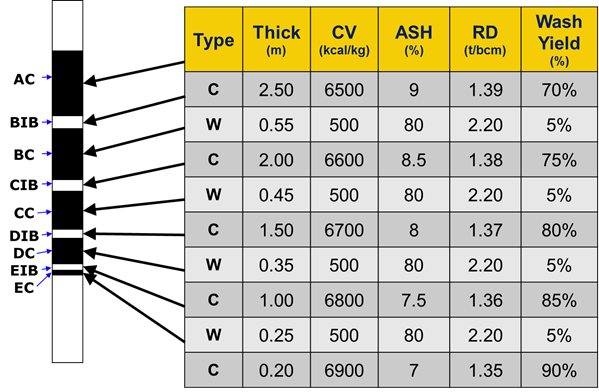Figure 1 – Sample hole

The usual rule of thumb which is likely to be applied in this case would be to waste ply EC as shown in Figure 2.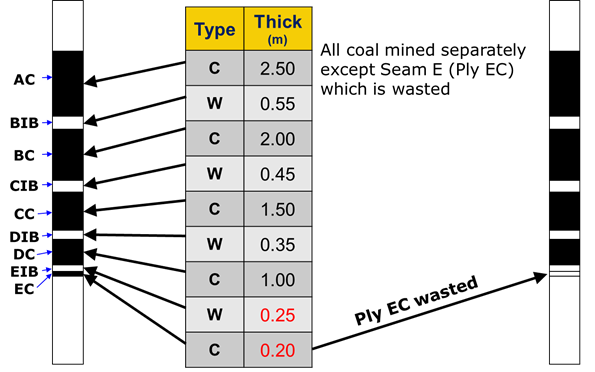Figure 2 – Ply EC wasted by application of rule of thumb

Using the same hole there are several alternative possibilities as presented in Figure 3.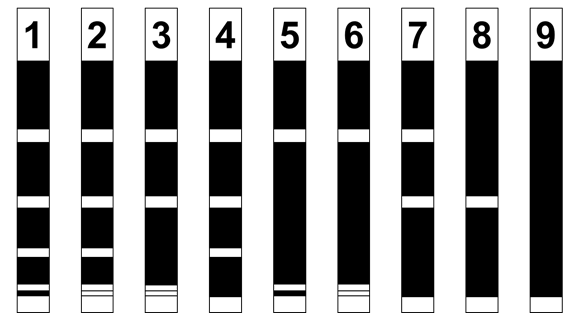Figure 3 – Some alternative possibilities

In the example given there are nine layers and 45 possible combinations.  Using a common rule of thumb there would be only one result, however considering that each layer may be mined as coal or waste there are in fact 90 possible combinations.  The simplest way of visualising the combinations is by way of a Pascal’s triangle as shown in Figure 4.  In this figure each possible combination is evaluated on the basis of being mined as ore or waste.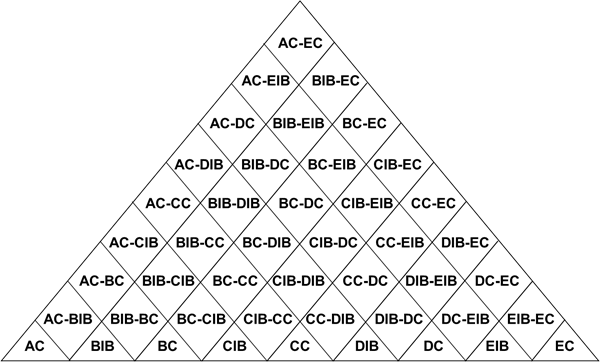Figure 4 – Pascal’s triangle of possible combinations

In Figure 4 each layer is suffixed with a “C” for “Coal” and “IB” for “Interburden”.  When considering the monetary value of each combination the following factors must be considered:

• Loss of ore at each interface
• Dilution at each interface
• Product yield or plant recovery
• Cost of mining as a function of thickness for both ore and waste
• Cost of processing including the handling of extra rejects or tailings

The monetary value for each possible combination is presented in Figure 5.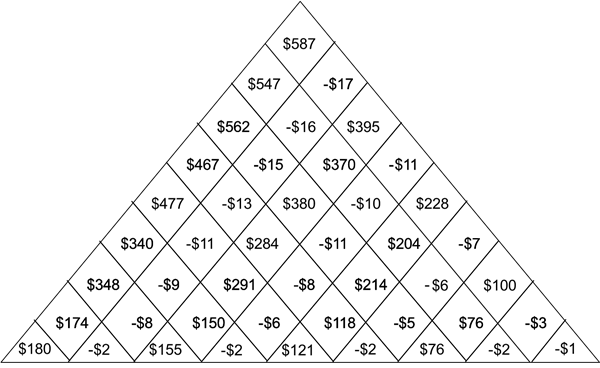Figure 5 – Monetary value of each possible combination

In Figure 5 the value of mining all the layers individually is the sum of the lowest level of values, i.e. \$180 - \$2 + \$155 - \$2 + \$121 - \$2 + \$76 - \$2 - \$1 = \$523.  Applying the rule of thumb in which the lowest two layers would be wasted would give a value of \$526, but there are a number of combinations which would result in higher values than this.  An example is presented in Figure 6.  For this example, the total value would be \$348 - \$2 + \$214 = \$560 which is approximately 6.5% better than result using the rule of thumb.  A quick examination of Figure 6 shows that there are two results which are even better than this, one of these being the value three steps down on the left-hand side which shows a total value of \$562.  The best result however is where all the layers are mined in a single pass.  In this case the total value is \$587 which is 11.6% better than the rule of thumb result.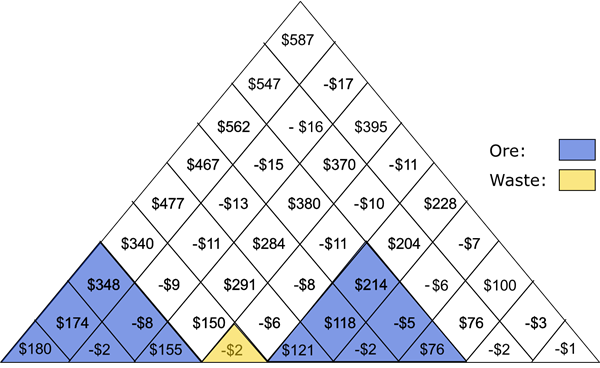Figure 6 – A selected possible combination

It is important to realize that this method cannot be properly applied to the drillhole data.  The reason for this is shown in Figure 7.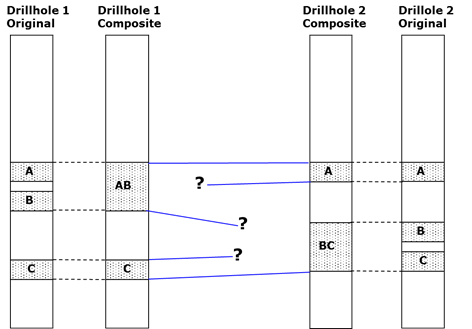Figure 7 – Method cannot be properly applied on a drillhole basis

Instead the method should be applied on a model basis as shown in Figure 8.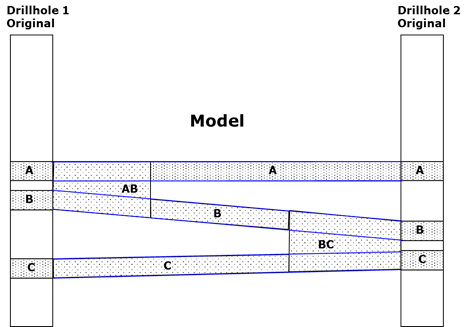Figure 8 – Method applied on a model basis

Following the compositing of plies, it will usually be found that various areas of the model have different composites.  This is illustrated in Figure 9.  In this example three sets of composites were created.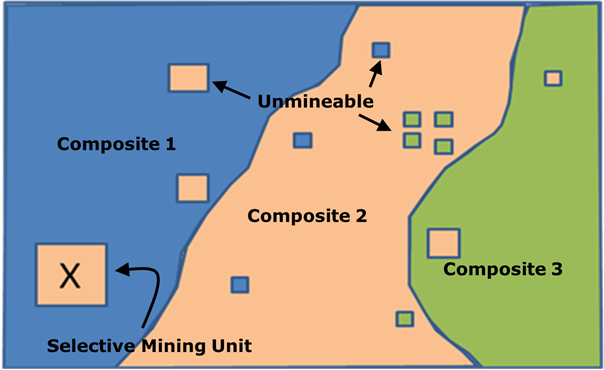Figure 9 – Geological composite model

In Figure 9 there are some areas within each area which are smaller than the minimum selective mining unit.  This means that it would be impractical to change the composite within this area.  Each of these smaller areas can either be:

1. Expanded to make it large enough to be mined separately or,
2. Removed and mined as per the surrounding composite.

It may be considered that a solution to this problem would be to simply use a model cell size equal to the size of the selective mining unit.  This solution however relies on predefining the origin and extent of each selective mining unit which would almost certainly produce a sub-optimal result.

ASEAMCO’s solution to this problem has been to list all solutions at each node as cumulative values and to subsequently use the floating cone optimization method with a cone truncated to the size of the selective mining unit to produce a set of seam composites each of which covers a practical mining area.

An example of the result following post-processing is presented in Figure 10.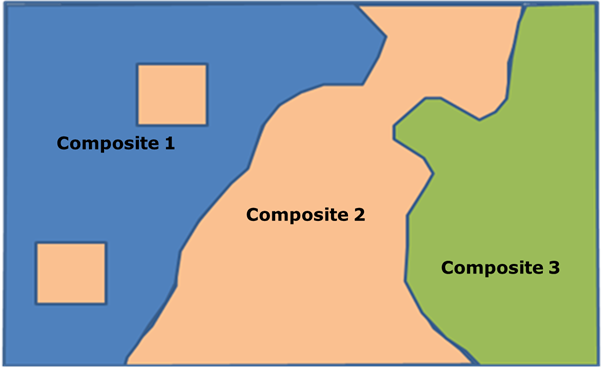Figure 10 – Result after post-processing

Real world results

ASEAMCO developed this application and used it to evaluate alternative strategies for a coal project in the Hunter Valley of New South Wales, Australia.  The final outcome resulted in:

• An increase in total coal recovery from 90.5% to 95.5%
• An increase in run-of-mine tonnage of 14.2%
• An increase of 5.9% in coal product tonnage
• A reduction of 9.1% in total operating cost per product tonne
• An increase in annual EBITDA of more than 7% without any change in equipment or manning

Although the coal processing plant yield reduced from 66.5% to 61.7%, the plant had excess throughput capacity and the additional plant operating costs were more than offset by the increased product output and reduced mining costs.

Other Applications

This method can be applied to any bedded deposit and can also be applied to underground mining where alternative roof and floor positions need to be considered.

Conclusions

• Data must be collected at sufficient resolution to allow compositing
• Rules of thumb are unreliable
• Simple compositing tends to produce unusable results
• Improvement in annual cash flow for mines with multiple seams or multiple coal plies that require compositing can be significant
• Can be applied to underground mining